# Mercer theorem

(diff) ← Older revision | Latest revision (diff) | Newer revision → (diff)

The bilinear series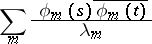of a Hermitian positive-definite continuous kernel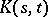on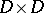(cf. Integral equation with symmetric kernel; Kernel of an integral operator), whereis the closure of a bounded domain in, converges absolutely and uniformly into. Here the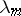are the characteristic numbers of the kerneland the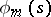are the corresponding orthonormalized eigen functions. If a kernelsatisfies the conditions of Mercer's theorem, then the integral operator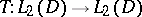,is nuclear (cf. Nuclear operator) and its tracecan be calculated by the formula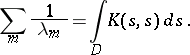Mercer's theorem can be generalized to the case of a bounded discontinuous kernel.

The theorem was proved by J. Mercer .

How to Cite This Entry:
Mercer theorem. Encyclopedia of Mathematics. URL: http://encyclopediaofmath.org/index.php?title=Mercer_theorem&oldid=11889
This article was adapted from an original article by V.B. Korotkov (originator), which appeared in Encyclopedia of Mathematics - ISBN 1402006098. See original article